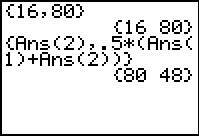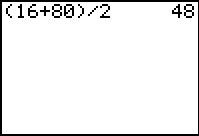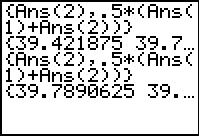# Activities

••• ##### Subject Area

• Math: Middle Grades Math: Algebraic Thinking

• ##### Author6-8

45 Minutes

• ##### Device
• TI-73 Explorer™
• TI-Navigator™

TI Connect™

## Average Sequence#### Activity Overview

In this activity, students will find the arithmetic mean between two consecutive numbers in a sequence to generate the next term and investigate the pattern that occurs.

#### Key Steps

•Students will solve the average sequence problem and share what they find. First, students find the arithmetic mean between two consecutive numbers in a sequence to generate the next term.

•Then, students use the list features and calculations to find subsequent terms in the sequence. This will help students generalize about what is happening and find the 100th term in the sequence.

•Finally, students look at a case where the first two elements in a sequence are decreasing to determine if the pattern holds.